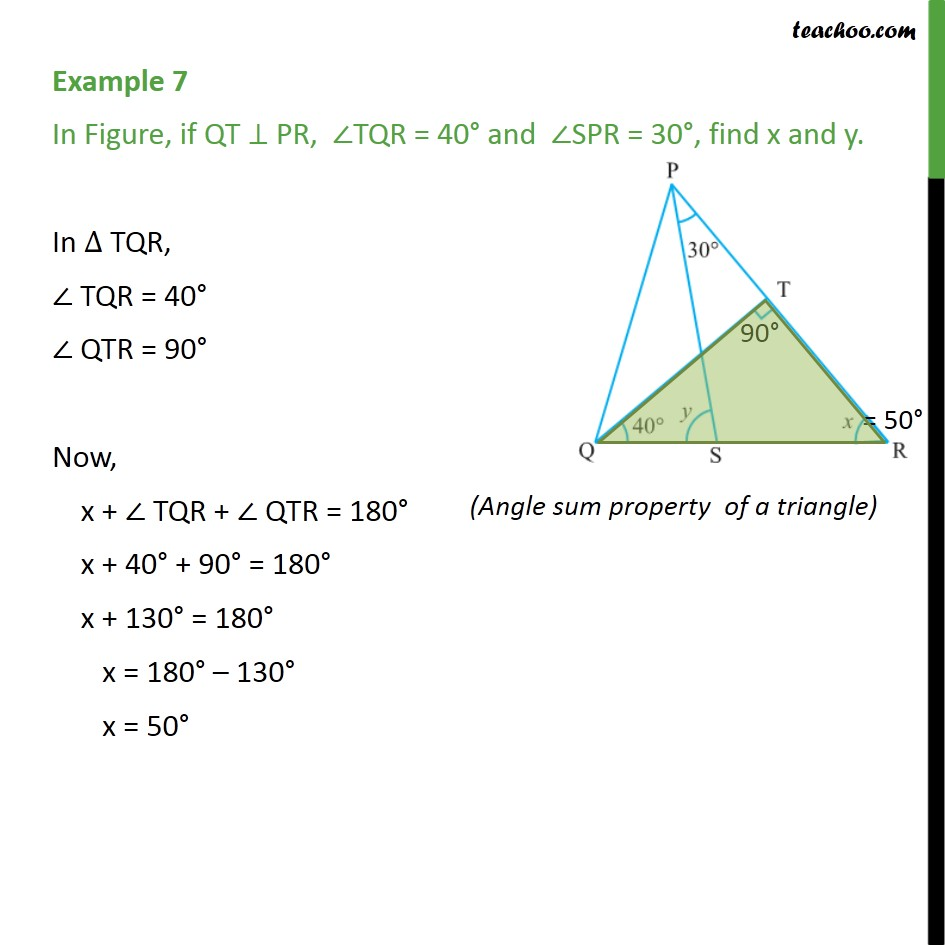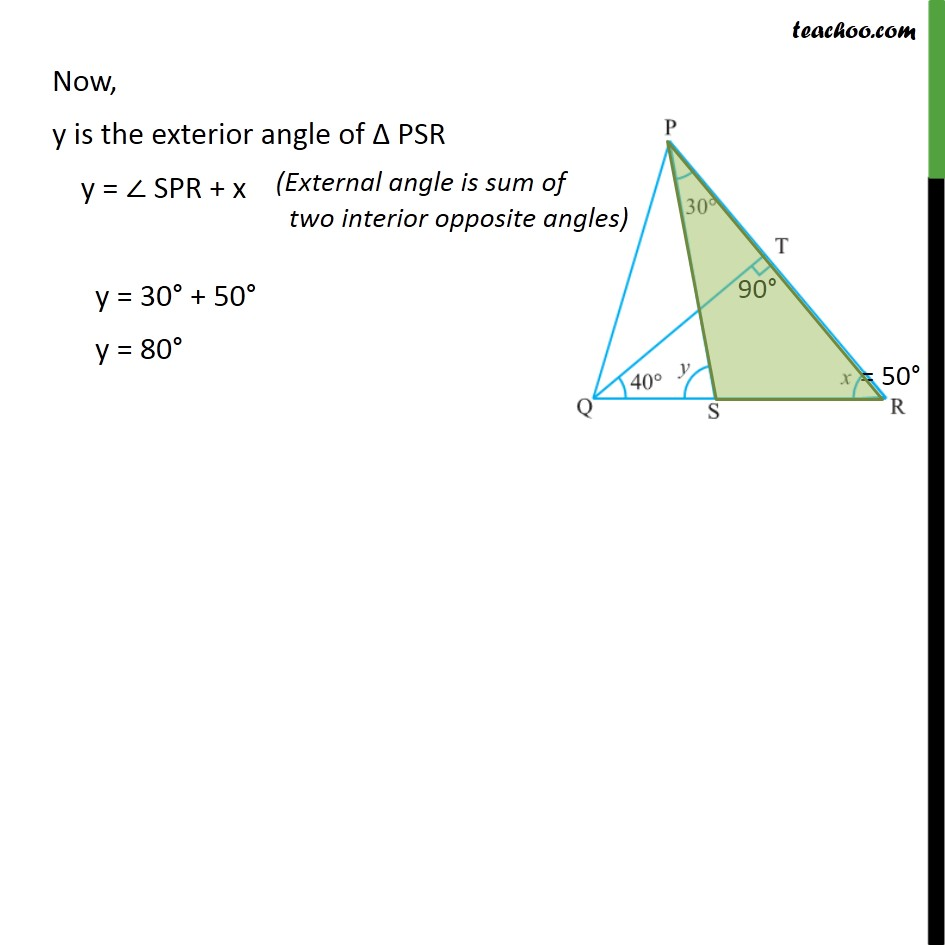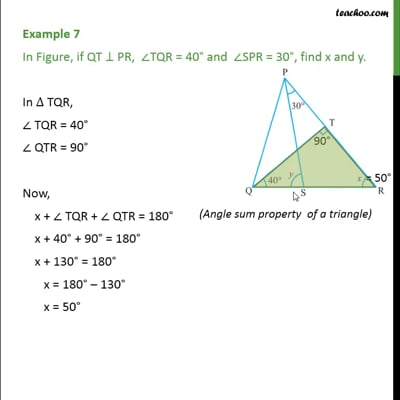Examples

Chapter 6 Class 9 Lines and Angles
Serial order wiseThis video is only available for Teachoo black users

Maths Crash Course - Live lectures + all videos + Real time Doubt solving!

### Transcript

Example 7 In Figure, if QT ⊥ PR, ∠TQR = 40° and ∠SPR = 30°, find x and y. In ∆ TQR, ∠ TQR = 40° ∠ QTR = 90° Now, x + ∠ TQR + ∠ QTR = 180° x + 40° + 90° = 180° x + 130° = 180° x = 180° – 130° x = 50° Now, y is the exterior angle of Δ PSR y = ∠ SPR + x y = 30° + 50° y = 80°